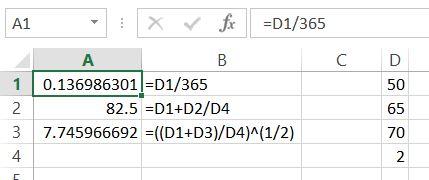## Entering Data in Excel

In this Excel lesson you will teach yourself how to enter data in Excel spreadsheet.For cells on a worksheet, you can enter data type:

• text,
• number,
• date,
• time

To indicate where to put the data appear, activate the cell (eg by clicking). Data is entered directly in the spreadsheet or above the working area in the input line in the formula bar.

To confirm the data, press the Enter key. To withdraw from making changes, you can press the Escape key.

## Entering text

Texts are both in the worksheet to record information (eg, name, name) and description of other data. By default, the text is aligned to the left side of the cell. If the text does not fit in the cell – extends to the empty cells in his right hand, or hiding under their content.

## Entering numbers

The numbers are usually the most important data entered into the worksheet. To be able to perform calculations on them, the program must interpret the contents of the cell as a number. At default settings, the number entered is aligned to the right edge of the cell.

## Entering formulas

To perform the calculation worksheet, enter a formula into a cell where you want to display the result.

The formula is a formula which is to calculate the value.

The formula contains, among others cell addresses from which to retrieve the data and the mathematical signs (operators).

## Enter a formula that adds together the contents of two cells

Enter a formula starts with entering the keyboard character = (equal to). Then click on the cell that is involved in the formula – the address is typed into a cell, enter a mathematical operation (eg + plus) and click on the second cell.

Formula should be ready to press the Enter key.

The basic operators of activities:

• + Add eg = A1 + B1,
• – Subtraction for example = A1-365,
• * (Asterisk) Multiplication eg = A5 * B12,
• / (slash) = B15/C20 division,
• ^ (Caret) exponentiation eg = A5 ^ 3,
• & (Ampersand) combination (concatenation) of texts.

The formula may include:

• addresses such as C10 = \$ B \$ 8 (address can be in any form – see the section on addressing)
• number eg = C20/365,
• text eg = “Ms / Mr” & B5 & “” & C5 (for example, when B5 and C5 is written the name),
• functions such as =SUM(C10: C20).

Activities in the formula are made in accordance with a mathematical sequence (eg, first multiply, then add). When dealing with the same priority (for example, multiplication and division) actions are executed from left to right formula. To force the order of operation is used parentheses, eg = ((A1 + A3) / B7-(B7-365) / 12) * 10%.

When you enter a formula in the cell displays the result of action, and the formula is visible on the canvas in the formula bar.

In the formula bar, you can also:

• enter and modify the formula,
• approve the formula by clicking on the Save button (looks like a hook).
• Formula can be copied and transferred to other cells.
• When you move the addresses appearing in the formula does not change.
• When copying, and using the drag handle fill addresses change depending on the addressing (see the section on addressing).
• Convenient form of copying is dragging content using fill handle (see subsection series data).

Entering and drag the formula.Sample formula:

=B4/365

=A1+B7/G34

=((D1+D3)/E3))^(1/2)

## Template

```Further reading: